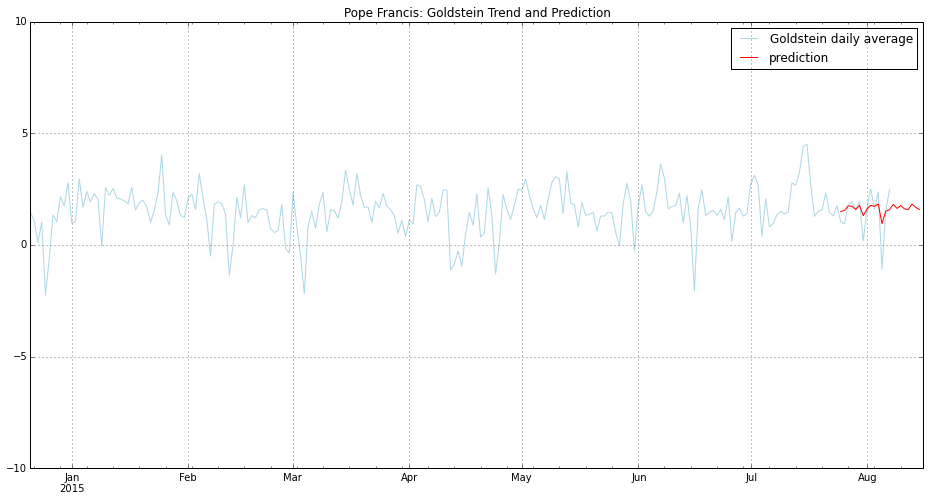# International Political Tele-Conferencing Equilibrium System (IPTCES) algorithm¶

The following is a public-facing walkthrough of the code used to for the predictive tool that powers @WorldLeaderTips. The styling of the out graph has been changed for this context.

In :
%pylab inline

Populating the interactive namespace from numpy and matplotlib

In :
import json
import random
import datetime as dt
from collections import deque

import numpy as np
import pandas as pd
from pandas import *
from pandas.io.json import json_normalize

import matplotlib.pylab
import matplotlib.pyplot as plt
import matplotlib.dates as mdates

import statsmodels.api as sm
from statsmodels.tsa import *

from bigquery import get_client

In :
tomorrow = str(dt.date.today())
twoweeksago = str(dt.date.today() - dt.timedelta(days = 14))
lastweek = dt.datetime.today() - dt.timedelta(days = 7)
nextweek = dt.date.today() + dt.timedelta(days = 8)
day_index = dt.datetime.today().weekday()

In :
# Initialize Google BigQuery client:
with open('/PATH/TO/settings.json', 'rb') as json_data:

client = get_client(settings['PROJECT_ID'], service_account=settings['SERVICE_ACCOUNT'], private_key=settings['KEY'], readonly=True)

In :
# Load the Goldstein suggstions table:

In :
leader_display_name = 'Pope Francis'
gdelt_search = "WHERE Actor1Name CONTAINS 'POPE' AND SQLDATE > 20130313"

date_start = '19790101'
date_end = ''.join(str(dt.date.today()).split('-'))

query_template = 'SELECT GLOBALEVENTID, SQLDATE, Actor1Name, Actor1CountryCode, GoldsteinScale, NumMentions FROM [gdelt-bq:full.events] {} AND SQLDATE>{} AND SQLDATE<{} IGNORE CASE;'
query = query_template.format(gdelt_search, date_start, date_end)

print 'Query: {}'.format(query)

Search for: Pope Francis
Query: SELECT GLOBALEVENTID, SQLDATE, Actor1Name, Actor1CountryCode, GoldsteinScale, NumMentions FROM [gdelt-bq:full.events] WHERE Actor1Name CONTAINS 'POPE' AND SQLDATE > 20130313 AND SQLDATE>19790101 AND SQLDATE<20150808 IGNORE CASE;

In :
filename = '{}.csv'.format(leader_display_name.replace(' ', '_'))

In :
try:
job_id, results = client.query(query, timeout=2000)
print '{} results'.format(str(len(results)))

if len(results) == 0:

df = json_normalize(results)
df = df.sort(columns='SQLDATE')
df.to_csv('{}'.format(filename), encoding='utf-8')
print 'Saved: {}'.format(filename)

except:
print 'ERROR: Timed out'

56788 results
Saved: Pope_Francis.csv

In :
df = pd.read_csv(filename, index_col='SQLDATE', parse_dates=True)
df.tail()

Out:
Unnamed: 0 Actor1CountryCode Actor1Name GLOBALEVENTID GoldsteinScale NumMentions
SQLDATE
2015-08-07 39523 NaN THE POPE 455865092 1.0 1
2015-08-07 39522 NaN THE POPE 455865091 1.0 1
2015-08-07 39521 NaN THE POPE 455865090 1.0 7
2015-08-07 39535 NaN THE POPE 455885123 1.9 10
2015-08-07 39619 NaN THE POPE 456037032 0.0 10
In :
# These steps incorporate the number of mentions a Goldstein score is associated with, reducing the impact of error in the event encoding, making the average better reflect the event's presence in the GDELT.
df['GoldMentions'] = df['GoldsteinScale'] * df['NumMentions']
goldstein = df.groupby([df.index.date]).agg({'GoldMentions': np.sum, 'NumMentions': np.sum})
goldstein['GoldAverage'] = goldstein['GoldMentions'] / goldstein['NumMentions']

In :
full_daterange = pd.date_range(start=min(df.index), end=max(df.index))

In :
# interpolate() takes care of days that do not have a entry / Goldstein score in GDELT:
goldstein = goldstein.reindex(full_daterange).interpolate(method='linear')

In :
# In case there is not enough historical data for the world leader:
if len(goldstein['GoldAverage']) < 230:
day_difference = 230 - len(goldstein['GoldAverage']) + 1

new_dates = [goldstein.index - dt.timedelta(days=x) for x in range(1, day_difference)]
new_dates = reversed(new_dates)

columns = ['NumMentions', 'GoldMentions', 'GoldAverage']
temp_data = {col: [None for x in range(1, day_difference)] for col in columns}
missing = pd.DataFrame(temp_data, index=new_dates, columns=columns)

temp_goldstein = missing.append(goldstein)
goldstein = temp_goldstein.fillna(method='bfill')

print 'Insufficient data, missing entries were backfilled.'
else:
print 'Sufficient data, backfilling was avoided.'

Sufficient data, backfilling was avoided.

In :
#Create two instances of the data, one to train the model...
training = pd.DataFrame(goldstein['GoldAverage'])
training.index = pd.to_datetime(training.index)
training.columns = ['Goldstein daily average']
print 'Test Sample {}\n'.format(training.tail())

# ... the other to create the plot:
plot_sample = pd.DataFrame(goldstein['GoldAverage'][-230:])
plot_sample.index = pd.to_datetime(plot_sample.index)
plot_sample.columns = ['Goldstein daily average']
print 'Plot Sample {}'.format(plot_sample.tail())

Test Sample             Goldstein daily average
2015-08-03                 1.599440
2015-08-04                 2.368912
2015-08-05                -1.088742
2015-08-06                 1.525023
2015-08-07                 2.479502

Plot Sample             Goldstein daily average
2015-08-03                 1.599440
2015-08-04                 2.368912
2015-08-05                -1.088742
2015-08-06                 1.525023
2015-08-07                 2.479502

In :
# Creates the forcasting model using Autoregressive Moving Average (ARMA):
tries = 0
success = False
while tries < 6 and success is False:
try:
model = sm.tsa.ARMA(training,(12, tries)).fit()
success = True
except:
tries += 1

if success is False:
print "NOT SUCCESSFUL"

print model

<statsmodels.tsa.arima_model.ARMAResultsWrapper object at 0x110ef2cd0>

In :
# Creates the prediction based on the proceeding two weeks through one week into the future:
prediction = model.predict(twoweeksago, str(nextweek), dynamic = False)

# The fitting of the prediction to the model:
prediction = prediction.shift(-1)

In :
def stir_the_pot(sample_range, st_dev):
mean = sample_range.mean()
random_suggestion = None

# Randomly suggest something either above or below the stagnant Goldstein range:
if random.randint(0,1) == 1:
else:

print 'new suggestion: {}'.format(random_suggestion)
return float(random_suggestion)

In :
# Based on the Prediction, determine the Suggestion:
sample_window = training[-60:]
standard_deviation = float(sample_window.std())
suggestion = None

if standard_deviation < 2.1 and random.randint(1,5) > 2:
# The leader has been stuck in a rut and is not honing in on Goldstein Score of '1', 3/5 chance of getting a new suggestion:
print 'Your actions are too stagnant!'
suggestion = stir_the_pot(sample_window, standard_deviation)

predicts = round(prediction.ix[tomorrow:str(nextweek)].mean(), 1)

else:
# Finds the average of the Goldstein scores for the coming week:
predicts = round(prediction.ix[tomorrow:str(nextweek)].mean(), 1)
suggestion = round(((predicts - 1) * -1), 1)

print 'suggestion: {}'.format(suggestion)

Your actions are too stagnant!
new suggestion: -5.4
suggestion: -5.4

In :
# From the possible suggestions for the score, find one at random:
prediction_text = random.choice(gsCodes.loc[predicts].values.split(';')).strip()
gsDescription   = random.choice(gsCodes.loc[suggestion].values.split(';')).strip()

In :
print '=================================================='
print "{}'s Forecast: {} {} ".format(leader_display_name, predicts, prediction_text)
print "{}'s Suggested score: {} ".format(leader_display_name, suggestion)

if predicts == 1.0:
gsDescription = "You're doing great. Stay the course."
else:
print "Suggested actions for the coming week:\n{}".format(gsDescription)

print '=================================================='

==================================================
Pope Francis's Forecast: 1.7 Make an apology
Pope Francis's Suggested score: -5.4
Suggested actions for the coming week:
Perform a non-military demonstration or walk out on something
==================================================

In :
plot_sample.plot(kind='line',
ylim=(-10,10),
figsize=(16,8),
color='lightblue')
prediction.plot(kind='line',
label='prediction',
legend=True,
color='red')

Out:
<matplotlib.axes.AxesSubplot at 0x11151a610>In [ ]: